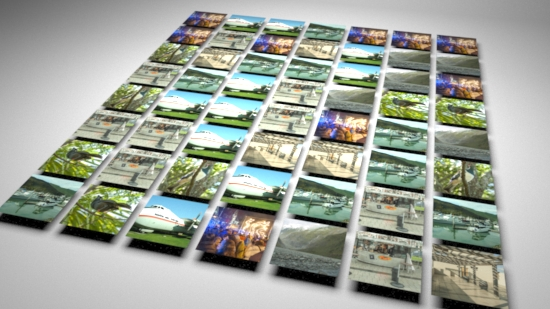### OSLMultiple Textures II

`Related Tutorial`

#### Introduction

This tutorial presents a two methods of applying 1 to 8 texture maps to multiple surfaces using a single material. Unlike the technique outlined in the tutorial,

Multiple Textures I

the two methods shown here DO NOT require each surface to be assigned a custom user attribute. Instead, the methods take advantage of an attribute that is automatically assigned to each surface by RfM. In a Rib file the attribute named "identifier:id" is of the form,

`    Attribute "identifier" "float id" `

The value of the attribute is used by the two shaders shown below to choose a texture that is read by the OSL `texture()` function.

#### Method 1: Cycling Texture Maps

The result of using the first shader, TexturePickerCycle.osl, on a grid of squares is shown in figure 1. The UI of the shader is shown in figure 2.Figure 1Figure 2

The shader uses simple arithmetic to calculate a value that is used to index into an array of texture paths.

 ```string textures = {tex1,tex2,tex3,tex4,tex5,tex6,tex7,tex8}; // Get the value of the objects id float id; if(getattribute("identifier:id", id)) { float rem = fmod(id/cycles, 1); int index = int(rem * 8); if(index >= 0 && index <= 8) { string texname = textures[index];```

For example, suppose the shader is evaluating a surface with an identifier:id of 13.
```rem = fmod(13.0/8, 1)    13.0/8 will be, 1.625    fmod(1.625, 1) hence rem will be 0.625    index = int(0.625 * 8)    0.625 * 8 will be, 5.0    int(5.0) hence index will be 5.```

Calculating "index" in this way ensures it will have a value in the range 0 to 7. Setting "cycles" to, say, 7 results in a semi-random distribution of textures.Figure 3

#### Method 2: Using Cellnoise to Choose Texture Maps

The result of using the second shader, TexturePickerNoise.osl, on a grid of squares is shown in figure 4.Figure 4

The shader uses a more computationally expensive technique to calculate a random value that is used to index into an array of texture paths. The use of OSL cellnoise() function is based on the method used by Pixar's PxrVisualizer plugin to produce random colors.

 ```string textures = {tex1,tex2,tex3,tex4,tex5,tex6,tex7,tex8}; // Get the value of the objects id float id; if(getattribute("identifier:id", id)) { color c = cellnoise( point(id, id + 7.0, id + 13.0) ); int index = int(c * 8); if(index >= 0 && index <= 8) { string texname = textures[index];```

Because cellnoise() returns repeatable noisy color values we can use either the red, green or blue component to generate an "index" value that is once again constrained to be in the range 0 to 7.